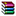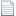# 心算任意年份的天干地支的方法

update: 修正笔误,增加口诀.2017-11-8

update: 增加从干支推算年份的方法. 2017-10-29

Update: 增加C#代码的实例 。2017-7-3天干地支心算法.png

=MID("甲乙丙丁戊已庚辛壬癸",MOD(A2-IF(A2>0,4,3),10)+1,1) & MID("子丑寅卯辰已午未申酉戌亥",MOD(A2-IF(A2>0,4,3),12)+1,1)

Function TGDZ(theYear As Integer) As String

Dim N As Integer, M1 As Integer, M2 As Integer

Application.Volatile

If theYear = 0 Then

MsgBox "年份不能为0,没有公元0年这一说法,开始即为公元1年"

TGDZ = "年份错误不能为0"

Exit Function

End If

N = theYear - IIf(theYear > 0, 4, 3)

M1 = (N Mod 10) + 10 * IIf((N Mod 10) < 0, 1, 0) + 1

M2 = (N Mod 12) + 12 * IIf((N Mod 12) < 0, 1, 0) + 1

TGDZ = Mid("甲乙丙丁戊已庚辛壬癸", M1, 1) & Mid("子丑寅卯辰已午未申酉戌亥", M2, 1)

End Function29除10余9, 9+1=10, 天干顺序中第10位即为癸。（实际上就是计算机中的取余）

29除12余5,5+1=6, 地支中第6位为巳。

13除10余3, 3+1=4, 天干中第4位为丁。

13除12余1, 1+1=2, 地支中第2位为丑。

-361-3=--364, 负数的求余不太好理解，可以加上整数倍个花甲（即60年天干地支1个循环）调整为正数再来看(不能直接去掉负号变成正数来求余)。

-364加上360得-4,还不够正数。再加一个60, 得56.

---------------------2017-10-29 更新--------------------------------

=1984

+MOD((12+FIND(LEFT(B2,1),"甲乙丙丁戊已庚辛壬癸")-FIND(RIGHT(B2,1),"子丑寅卯辰已午未申酉戌亥")),12)*10/2+

FIND(LEFT(B2,1),"甲乙丙丁戊已庚辛壬癸")-1

=-57+

MOD((12+FIND(LEFT(B2,1),"甲乙丙丁戊已庚辛壬癸")-FIND(RIGHT(B2,1),"子丑寅卯辰已午未申酉戌亥")),12)*5

+FIND(LEFT(B2,1),"甲乙丙丁戊已庚辛壬癸")-12018-1-31 最近学习matlab,补充个用m语言写的函数.TGDZ(matlab).rar

C#的小程序,CMD下面运行TGDZcalc.rar

http://blog.sciencenet.cn/blog-1213210-1026674.html

## 相关博文

GMT+8, 2019-8-23 19:22

Powered by ScienceNet.cn

Copyright © 2007- 中国科学报社